Author: Oscar Cronquist Article last updated on April 29, 2022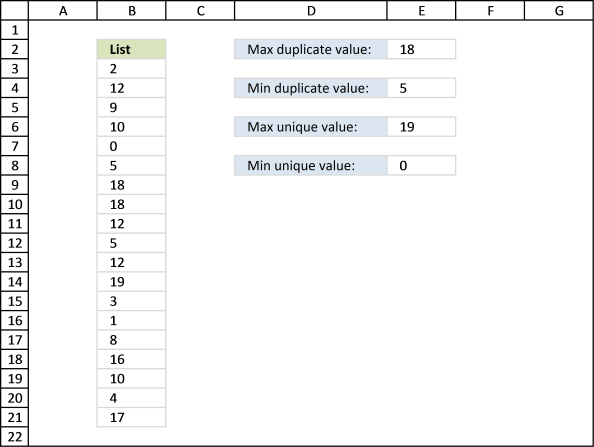## 1. Extract the largest duplicate number

Question: How do I get the largest and smallest unique and duplicate value?

The image below shows you a list of numbers in column B (\$B\$3:\$B\$21).Max duplicate value, array formula in E2:

The largest number in the list above is 19 but it is a unique number meaning it exists only once. Number 18 is the largest duplicate number, 18 is in cell B9 and B10.

=MAX(IF(COUNTIF(\$B\$3:\$B\$21,\$B\$3:\$B\$21)>1,\$B\$3:\$B\$21,))

To enter an array formula, type the formula in a cell then press and hold CTRL + SHIFT simultaneously, now press Enter once. Release all keys.

The formula bar now shows the formula with a beginning and ending curly bracket telling you that you entered the formula successfully. Don't enter the curly brackets yourself.

### 1.1 Explaining formula in cell E2

#### Step 1 - Count the frequency of each value

The COUNTIF function counts values based on a condition or criteria, in this case, it counts all values in the cell range.

COUNTIF(\$B\$3:\$B\$21,\$B\$3:\$B\$21)

becomes

COUNTIF({2; 12; 9; 10; 0; 5; 18; 18; 12; 5; 12; 19; 3; 1; 8; 16; 10; 4; 17},{2; 12; 9; 10; 0; 5; 18; 18; 12; 5; 12; 19; 3; 1; 8; 16; 10; 4; 17})

and returns

{1; 3; 1; 2; 1; 2; 2; 2; 3; 2; 3; 1; 1; 1; 1; 1; 2; 1; 1}.

#### Step 2 - Extract duplicate numbers

The IF function returns the row number if cell is a duplicate. FALSE returns "" (nothing).

IF(COUNTIF(\$B\$3:\$B\$21,\$B\$3:\$B\$21)>1,\$B\$3:\$B\$21,)

becomes

IF({1; 3; 1; 2; 1; 2; 2; 2; 3; 2; 3; 1; 1; 1; 1; 1; 2; 1; 1}>,\$B\$3:\$B\$21,)

becomes

IF({1; 3; 1; 2; 1; 2; 2; 2; 3; 2; 3; 1; 1; 1; 1; 1; 2; 1; 1}>,{2; 12; 9; 10; 0; 5; 18; 18; 12; 5; 12; 19; 3; 1; 8; 16; 10; 4; 17},)

and returns

{0; 12; 0; 10; 0; 5; 18; 18; 12; 5; 12; 0; 0; 0; 0; 0; 10; 0; 0}.

#### Step 3 - Return largest value

The MAX function returns the maximum value from a cell range or array.

MAX(IF(COUNTIF(\$B\$3:\$B\$21,\$B\$3:\$B\$21)>1,\$B\$3:\$B\$21,))

becomes

MAX({0; 12; 0; 10; 0; 5; 18; 18; 12; 5; 12; 0; 0; 0; 0; 0; 10; 0; 0})

and returns 18 in cell E2.

## 2. Extract the largest duplicate number - Excel 365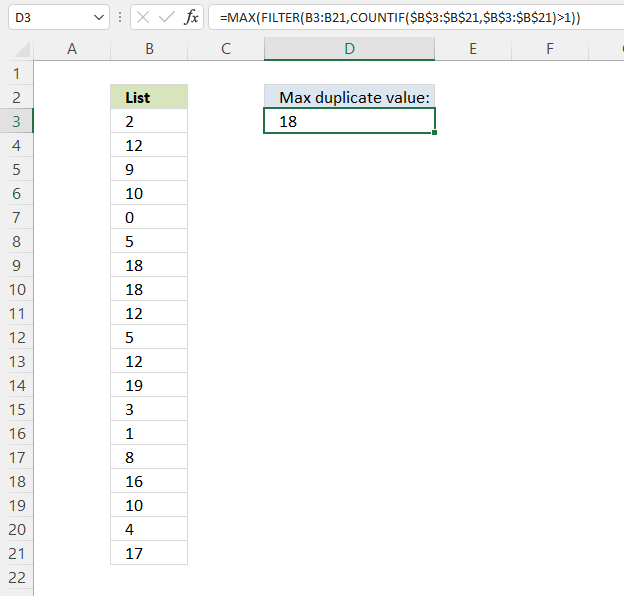Formula in cell D3:

=MAX(FILTER(B3:B21,COUNTIF(\$B\$3:\$B\$21,\$B\$3:\$B\$21)>1))

### 2.1 Explaining formula

#### Step 1 - Count each item

The COUNTIF function calculates the number of cells that meet a given condition.

COUNTIF(rangecriteria)

COUNTIF(\$B\$3:\$B\$21, \$B\$3:\$B\$21)

returns

{1; 3; 1; 2; 1; 2; 2; 2; 3; 2; 3; 1; 1; 1; 1; 1; 2; 1; 1}.

#### Step 2 - Check if larger than one meaning it is a duplicate

The larger than sign is a logical operator that lets you find numbers larger than a given condition. In this case, if larger than one means it is a duplicate.

COUNTIF(\$B\$3:\$B\$21,\$B\$3:\$B\$21)>1

becomes

{1; 3; 1; 2; 1; 2; 2; 2; 3; 2; 3; 1; 1; 1; 1; 1; 2; 1; 1}>1

and returns

{FALSE; TRUE; FALSE; TRUE; FALSE; TRUE; TRUE; TRUE; TRUE; TRUE; TRUE; FALSE; FALSE; FALSE; FALSE; FALSE; TRUE; FALSE; FALSE}

#### Step 3 - Filter duplicates

The FILTER function lets you extract values/rows based on a condition or criteria.

FILTER(arrayinclude, [if_empty])

FILTER(B3:B21, COUNTIF(\$B\$3:\$B\$21, \$B\$3:\$B\$21)>1)

becomes

FILTER({2; 12; 9; ... ; 17}, {FALSE; TRUE; FALSE; ... ; FALSE})

and returns

{12; 10; 5; 18; 18; 12; 5; 12; 10}.

#### Step 4 - Get largest value

The MAX function gets the largest number in a cell range or array.

MAX(number1, [number2], ...)

MAX(FILTER(B3:B21,COUNTIF(\$B\$3:\$B\$21,\$B\$3:\$B\$21)>1))

becomes

MAX({12; 10; 5; 18; 18; 12; 5; 12; 10})

and returns 18.

## 3. Extract the smallest duplicate number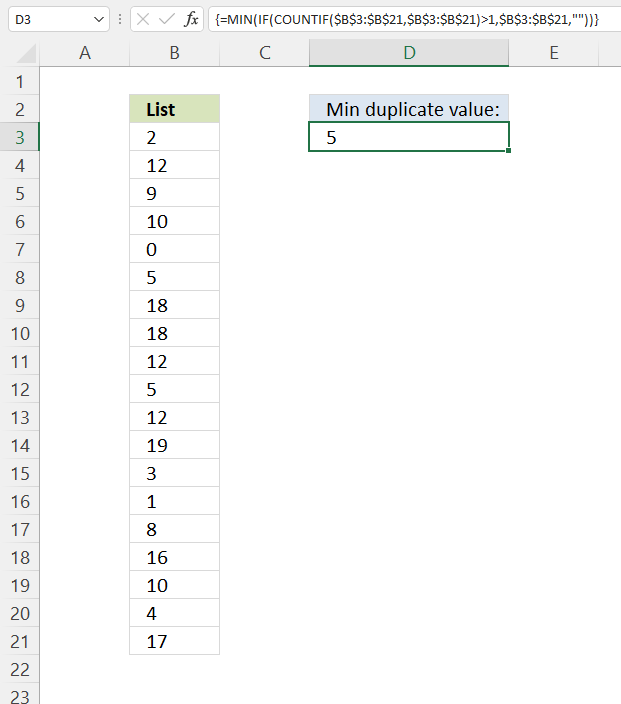Formula in cell

The smallest number in the list above is 0 but it is a unique number meaning it exists only once. Number 5 is the smallest duplicate number, 18 is in cell B8 and B12.

=MIN(IF(COUNTIF(\$B\$3:\$B\$21, \$B\$3:\$B\$21)>1, \$B\$3:\$B\$21, ""))

This formula is almost identical to the formula in section 1, only MAX function is replaced with the MIN function. See the formula explanation above in section 1.

## 4. Extract the smallest duplicate number - Excel 365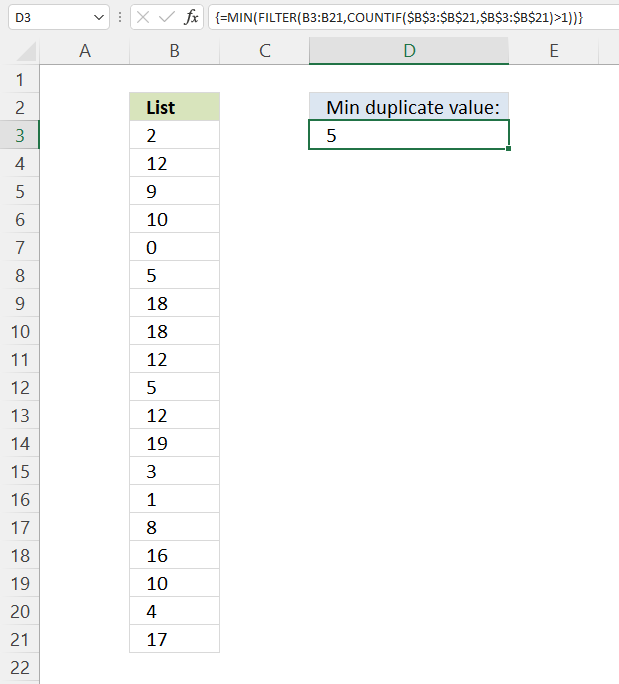Formula in cell D3:

=MIN(FILTER(B3:B21,COUNTIF(\$B\$3:\$B\$21,\$B\$3:\$B\$21)>1))

This formula is almost identical to the formula in section 2, only the MAX function is replaced with the MIN function. See the formula explanation above in section 2.

## 5. Extract the largest unique number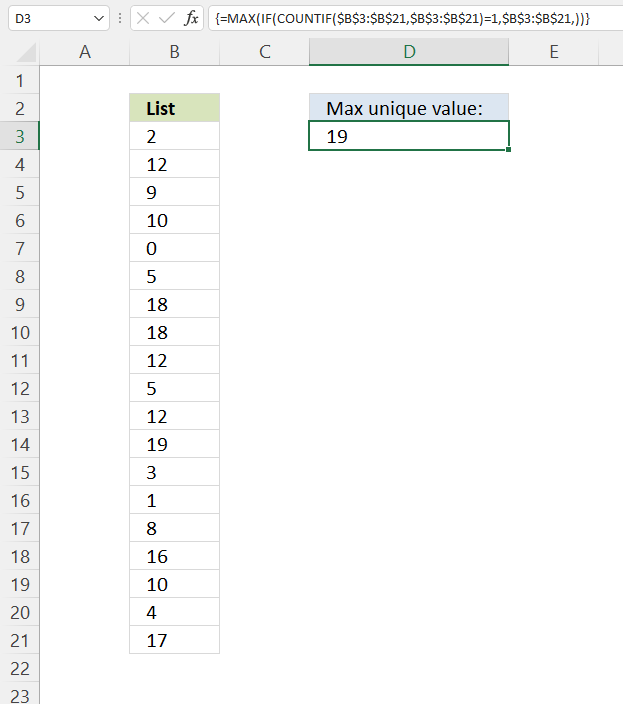Max unique value, formula in D3:

The largest unique number in the list above is 19.

=MAX(IF(COUNTIF(\$B\$3:\$B\$21, \$B\$3:\$B\$21)=1, \$B\$3:\$B\$21, ))

### 5.1 Explaining formula

#### Step 1 - Count values

The COUNTIF function calculates the number of cells that meet a given condition.

COUNTIF(rangecriteria)

COUNTIF(\$B\$3:\$B\$21, \$B\$3:\$B\$21)

becomes

COUNTIF({2; 12; 9; ... ; 17}, {2; 12; 9; ... ; 17})

and returns

{1; 3; 1; 2; 1; 2; 2; 2; 3; 2; 3; 1; 1; 1; 1; 1; 2; 1; 1}.

#### Step 2 - Check if a number is one

A value equal to one must be a unique value, it exists only once. The equal sign is a logical operator that lets you compare values in an Excel formula.

COUNTIF(\$B\$3:\$B\$21, \$B\$3:\$B\$21)=1

becomes

{1; 3; 1; 2; 1; 2; 2; 2; 3; 2; 3; 1; 1; 1; 1; 1; 2; 1; 1}=1

and returns

{TRUE; FALSE; TRUE; FALSE; TRUE; FALSE; FALSE; FALSE; FALSE; FALSE; FALSE; TRUE; TRUE; TRUE; TRUE; TRUE; FALSE; TRUE; TRUE}.

#### Step 3 - Evaluate IF function

IF(COUNTIF(\$B\$3:\$B\$21, \$B\$3:\$B\$21)=1, \$B\$3:\$B\$21, )

becomes

IF({TRUE; FALSE; TRUE; ... ; TRUE}, {2; 12; 9; ... ; 17}, )

and returns

{2; 0; 9; 0; 0; 0; 0; 0; 0; 0; 0; 19; 3; 1; 8; 16; 0; 4; 17}.

#### Step 4 - Get the largest number in the array

The MAX function gets the largest number in a cell range or array.

MAX(number1, [number2], ...)

MAX(IF(COUNTIF(\$B\$3:\$B\$21, \$B\$3:\$B\$21)=1, \$B\$3:\$B\$21, ))

becomes

MAX({2; 0; 9; 0; 0; 0; 0; 0; 0; 0; 0; 19; 3; 1; 8; 16; 0; 4; 17})

and returns 19.

## 6. Extract the largest unique number - Excel 365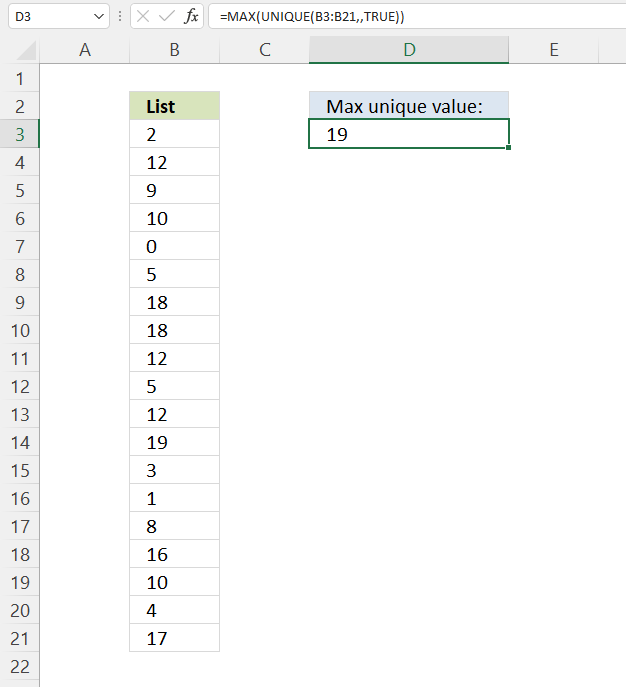Formula in cell D3:

=MAX(UNIQUE(B3:B21, , TRUE))

### Explaining formula

#### Step 1 - Extract unique numbers in cell range B3:B21

The UNIQUE function lets you extract both unique and unique distinct values.

UNIQUE(array, [by_col], [exactly_once])

UNIQUE(B3:B21, , TRUE)

becomes

UNIQUE({2; 12; 9; ... ; 17}, , TRUE)

and returns

{2; 9; 0; 19; 3; 1; 8; 16; 4; 17}

#### Step 2 - Get largest number in array

The MAX function gets the largest number in a cell range or array.

MAX(number1, [number2], ...)

MAX(UNIQUE(B3:B21, , TRUE))

becomes

MAX({2; 9; 0; 19; 3; 1; 8; 16; 4; 17})

and returns 19.

## 7. Extract the smallest unique number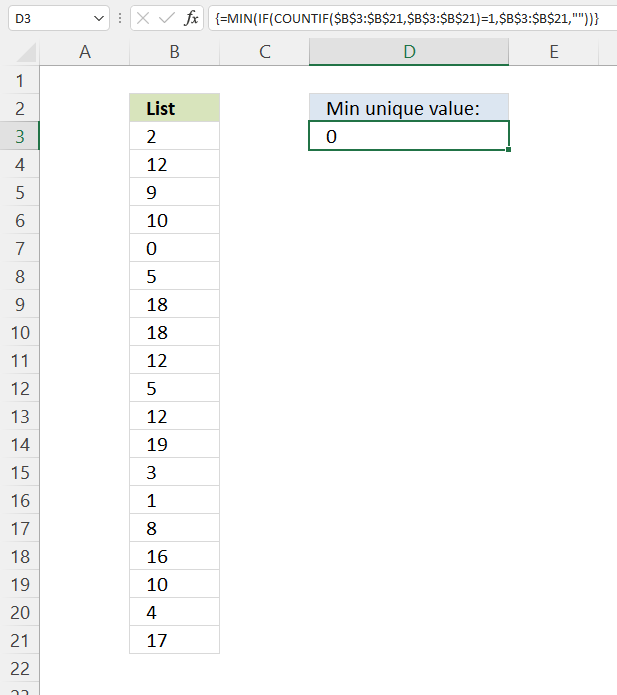Min unique value, formula in E8:

The smallest unique number in the list above is 0.

=MIN(IF(COUNTIF(\$B\$3:\$B\$21, \$B\$3:\$B\$21)=1, \$B\$3:\$B\$21, ""))

This formula is almost identical to the formula in section 5, only the MAX function is replaced with the MIN function. See the formula explanation above in section 5.

## 8. Extract the smallest unique number - Excel 365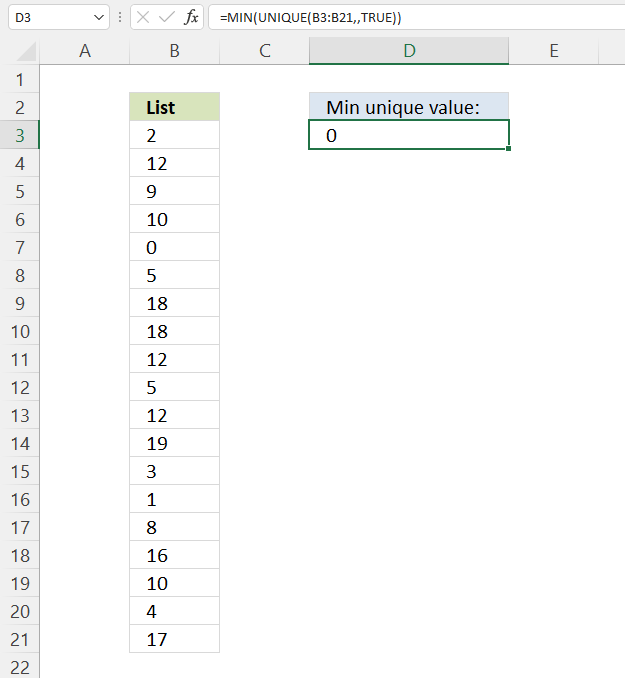Excel 365 dynamic array formula in cell D3:

=MIN(UNIQUE(B3:B21,,TRUE))

This formula is almost identical to the formula in section 6, only the MAX function is replaced with the MIN function. See the formula explanation above in section 6.

### Get the Excel fileMin-and-max-unique-and-duplicate-valuesv2.xlsx